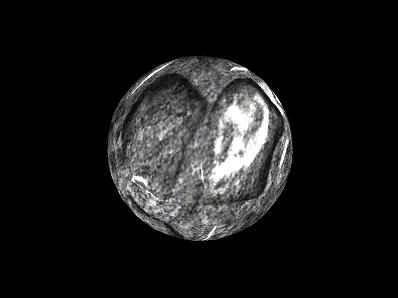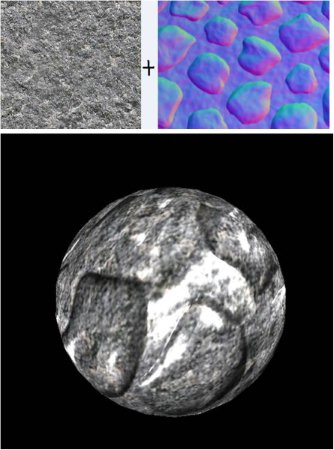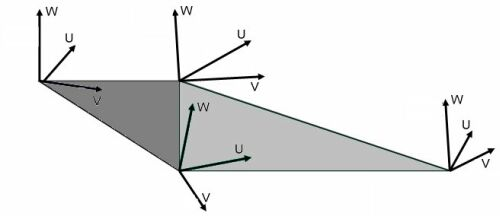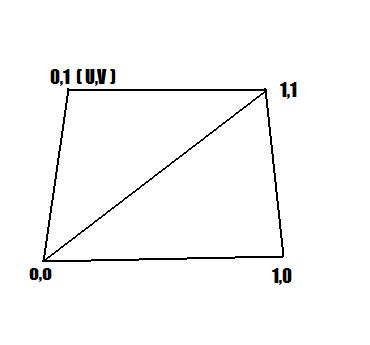## 切线空间V = W×U

texture ColorMap;

sampler ColorMapSampler = sampler_state
{
Texture = <ColorMap>; // sets our sampler to use ColorMap
MinFilter = Linear; // enabled trilinear filtering for this texture
MagFilter = Linear;
MipFilter = Linear;
}; 

technique NormalMapping
{
pass P0
{
}
} 

Ok，现在我们已经做好使用纹理的准备了！

float4 Color;

Color = tex2D( ColorMapSampler, Tex );
// Tex 是pixel shader的输入，它来自于vertex shader的输出，就是纹理坐标。float4x4 matWorldViewProj ;
float4x4 matWorld ;
float3 vecLightDir ;
float3 vecEye ; 

texture ColorMap;
sampler ColorMapSampler = sampler_state
{
Texture = <ColorMap>;
MinFilter = Linear;
MagFilter = Linear;
MipFilter = Linear;
};

texture NormalMap;
sampler NormalMapSampler = sampler_state
{
Texture = <NormalMap>;
MinFilter = Linear;
MagFilter = Linear;
MipFilter = Linear;
}; 

struct OUT
{
float4 Pos : POSITION;
float2 Tex : TEXCOORD0;
float3 Light :TEXCOORD1;
float3 View : TEXCOORD2;
}; 

OUT VS(float4 Pos : POSITION, float2 Tex : TEXCOORD, float3 N : NORMAL, float3 T : TANGENT )
{
OUT Out = (OUT)0;
Out.Pos = mul(Pos, matWorldViewProj);
float3x3 worldToTangentSpace;
worldToTangentSpace = mul(T, matWorld);
worldToTangentSpace = mul(cross(T, N), matWorld);
worldToTangentSpace = mul(N, matWorld);
Out.Tex = Tex;
float4 PosWorld = mul(Pos, matWorld);
Out.Light = mul(worldToTangentSpace, vecLightDir); // L
Out.View = mul(worldToTangentSpace, vecEye - PosWorld.xyz); // V return Out;
} 

float4 PS(float2 Tex: TEXCOORD0, float3 L : TEXCOORD1, float3 V :TEXCOORD2) : COLOR
{
float4 Color = tex2D(ColorMapSampler, Tex);
float3 N =(2 * (tex2D(NormalMapSampler, Tex)))- 1.0;
float3 LightDir = normalize(L); // L
float3 ViewDir = normalize(V); // V
float D = saturate(dot(N, LightDir));
float3 R = normalize(2 * D * N - LightDir); // R
float S = min(pow(saturate(dot(R, ViewDir)), 3), Color.w);
return 0.2 * Color + Color * D + S;
} 

float3 N =(2 * (tex2D(NormalMapSampler, Tex)))- 1.0;

technique NormalMapping
{
pass P0
{
}
}

Texture2D normalMap ;

object.colorMap = Content.Load<Texture2D>("stone");
normalMap = Content.Load<Texture2D>("normal"); 

effect.Parameters["ColorMap"].SetValue(colorMap);
effect.Parameters["NormalMap"].SetValue(normalMap); 

## 练习

1．改变不同的colormaps看看结果。

2．尝试不同的模型，比如一个立方体用于创建一块砖墙或石墙。